# vs.eyeandcontacts.com

## Chapter 15 Introduction to Graphs Exercise 15.2

Question 1: Plot the following points on a graph sheet. Verify if they lie on a line
a) A(4, 0), B(4, 2), C(4, 6), D(4, 2.5)
b) P(1, 1), Q(2, 2), R(3, 3), S(4, 4)
c) K(2, 3), L(5, 3), M(5, 5), N(2, 5)

a)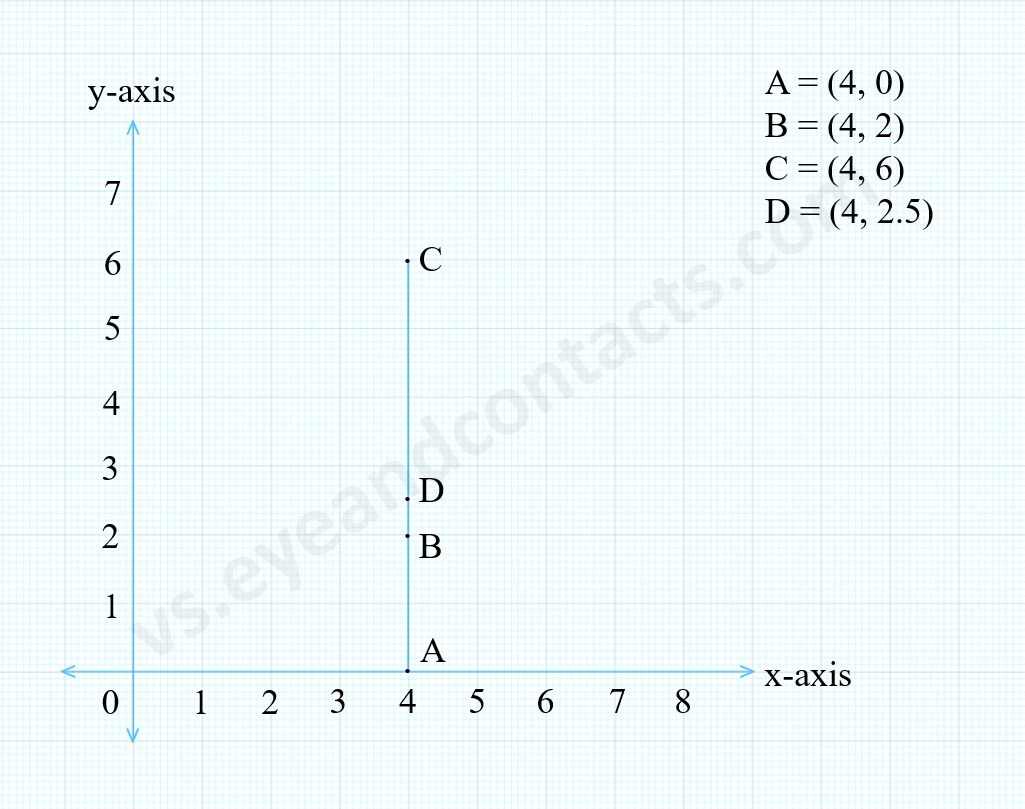Therefore, ABCD lie on a line.

b)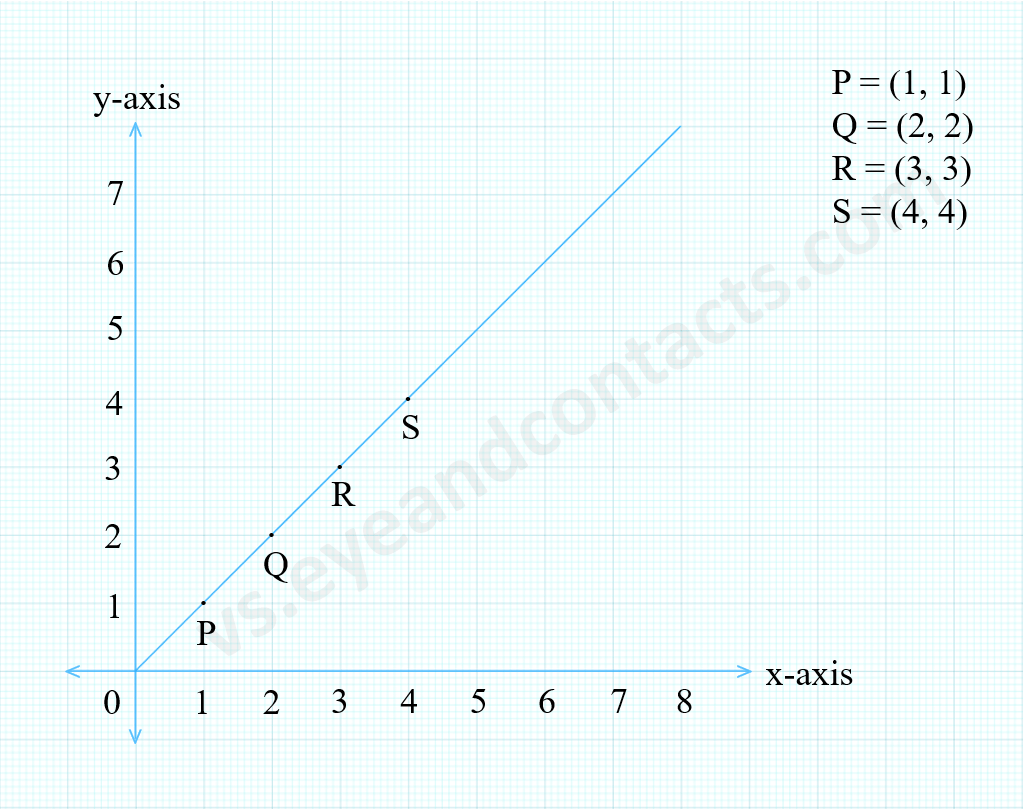Therefore, PQRS lie on a line.

c)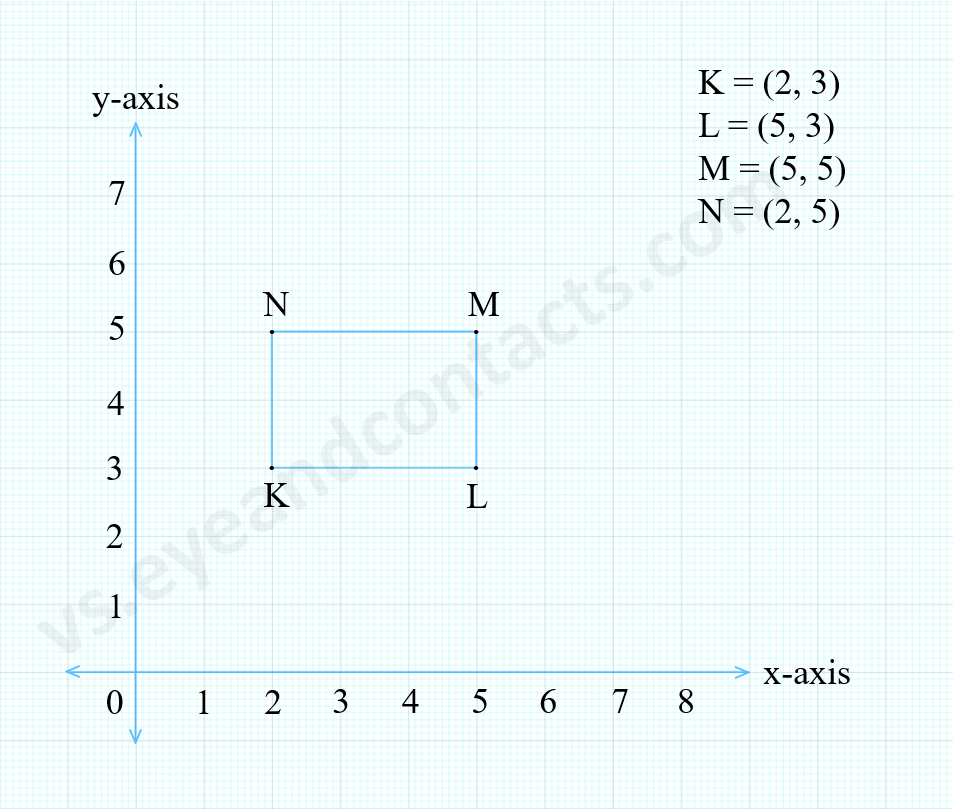Therefore, KLMN don’t lie on a line.

Question 2: Draw the line passing through (2, 3) and (3, 2). Find the coordinates of the points at which this line meets the x-axis and y-axis.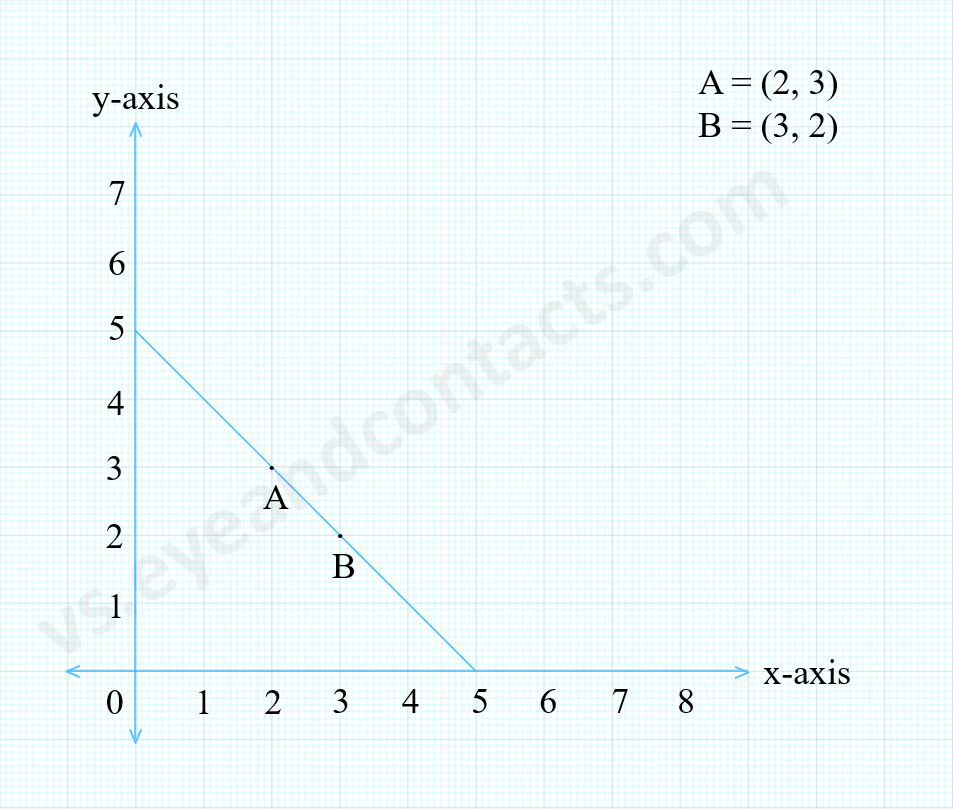x-axis at (5, 0)
y-axis at (0, 5)
(5, 5) is the coordinate of the points at which this line meets x-axis and y-axis.

Question 3: Write the coordinates of the vertices of each of these adjoining figures.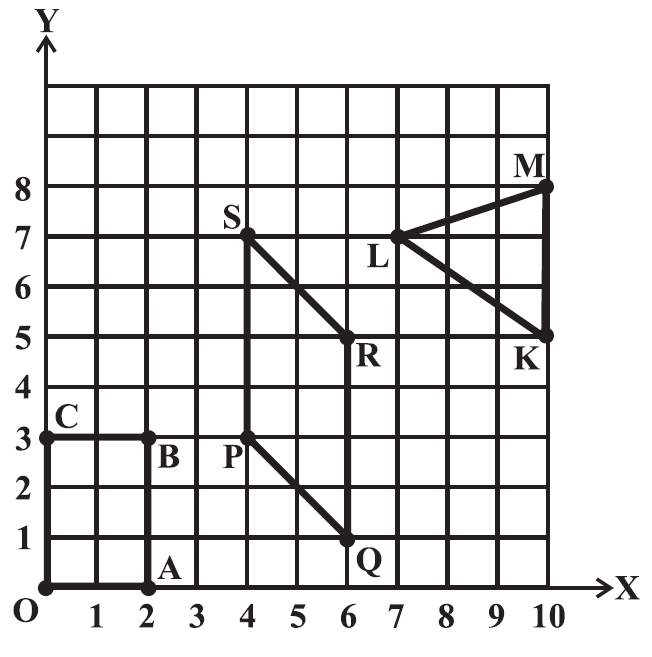O(0, 0)
A(2, 0)
B(2, 3)
C(0, 3)
P(4, 3)
Q(6, 1)
R(6, 5)
S(4, 7)
K(10, 5)
L(7, 7)
M(10, 8)

Question 4: State whether True or False. Correct that are false.
i) A point whose x coordinate is zero and y-coordinate is non-zero will lie on the y-axis.
ii) A point whose y coordinate is zero and x-coordinate is 5 will lie on y-axis.
iii) The coordinates of the origin are (0, 0).